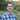# Create Random Django Compatible Usernames in Objective-C

June 28, 2012

I’m working on an app right now that lets anonymous users update data. Only I don’t quite want them to be anonymous. I want to be able to keep track of “who” data belongs too in order to provide individual service. So I needed to create unique usernames to register with the server. I extended the NSString class and add a UUID method. Problem with that is the resulting string was too long for Django. So I added another method to convert it to a base 64 ASCII string of alphanumeric characters (and two special characters). Here’s my implementation file.

``````#import "NSString+UUID.h"

@implementation NSString (UUID)

+ (NSString *)Base64Encode:(NSData *)data{
//Point to start of the data and set buffer sizes
int inLength = [data length];
int outLength = ((((inLength * 4)/3)/4)*4) + (((inLength * 4)/3)%4 ? 4 : 0);
const char *inputBuffer = [data bytes];
char *outputBuffer = malloc(outLength);
outputBuffer[outLength] = 0;

//64 digit code
static char Encode[] = "ABCDEFGHIJKLMNOPQRSTUVWXYZabcdefghijklmnopqrstuvwxyz0123456789_-";

//start the count
int cycle = 0;
int inpos = 0;
int outpos = 0;
char temp;

//Pad the last to bytes, the outbuffer must always be a multiple of 4
outputBuffer[outLength-1] = '=';
outputBuffer[outLength-2] = '=';

while (inpos < inLength){
switch (cycle) {
case 0:
outputBuffer[outpos++] = Encode[(inputBuffer[inpos]&0xFC)>>2];
cycle = 1;
break;
case 1:
temp = (inputBuffer[inpos++]&0x03)<<4;
outputBuffer[outpos] = Encode[temp];
cycle = 2;
break;
case 2:
outputBuffer[outpos++] = Encode[temp|(inputBuffer[inpos]&0xF0)>> 4];
temp = (inputBuffer[inpos++]&0x0F)<<2;
outputBuffer[outpos] = Encode[temp];
cycle = 3;
break;
case 3:
outputBuffer[outpos++] = Encode[temp|(inputBuffer[inpos]&0xC0)>>6];
cycle = 4;
break;
case 4:
outputBuffer[outpos++] = Encode[inputBuffer[inpos++]&0x3f];
cycle = 0;
break;
default:
cycle = 0;
break;
}
}

NSString *datastring = [NSString stringWithUTF8String:outputBuffer];
free(outputBuffer);

return datastring;
}

+ (NSString *)uuid
{
NSString *uuidString = nil;
CFUUIDRef uuid = CFUUIDCreate(NULL);
if (uuid) {
CFUUIDBytes bytes = CFUUIDGetUUIDBytes(uuid);
NSData *uidBytes = [NSData dataWithBytes:&bytes length:sizeof(bytes)];

uuidString = [self Base64Encode:uidBytes];
CFRelease(uuid);
}
return [uuidString substringToIndex:[uuidString length] - 2];
}

@end``````

Working well for me. Now I need to look into getting Django TastyPie APIs setup to use this.

Base 64 implementation credit goes to StackOverflow. I’m afraid that I don’t remember where I pulled the UUID method from, though I have tweaked it a fair amount since.Written by Luke Ryan Jernejcic who lives and works in Austin Texas building useful things. Follow him on Twitter